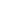# Sketch of a Three-Part Lesson

#### Before: Getting Started: 10–15 minutes

The purpose of this preliminary part of the lesson is to get the students to be cognitively prepared for the lesson problem by having them think about ideas and strategies they have learned and used before. The teacher organizes a revisit to a concept, procedure or strategy related to the lesson’s learning goal. The revisit might be a class discussion of the previous lesson problem, students demonstrating methods or strategies that were developed to solve previous problems, or students solving a smaller problem that evokes prior knowledge, skill and strategies.

#### During: Working on It: 30–40 minutes

For this part of the lesson, the students are actively solving the problem. They work in small groups, in pairs, or individually to solve a problem and record the mathematical thinking they used to develop solutions. Students develop independence and confidence by choosing the methods, strategies and concrete materials they will use, as well as ways to record their solutions. When students are given sufficient time to solve a problem, they learn to develop perseverance and come to expect that solutions will not be immediately apparent and that it takes time to solve a math problem.

While the students are making a plan and carrying it out to solve the lesson problem, the teacher circulates, making observations about the ways students are interacting and taking note of the mathematical models of representation, methods, strategies and mathematical language the students use to develop their solutions. If students are stuck, the teacher might pose questions to provoke further thinking or have other students explain their plan for solving the problem.

#### After: Consolidation and Practice: 10–15 minutes

In this phase, the teacher strategically co-ordinates student sharing of solutions to the lesson problem, using a mathematical instructional strategy like bansho or math congress or a gallery walk. By using such a strategy, the teacher can facilitate a whole-class discussion whereby students explain the mathematics in their solutions, methods, and strategies and discern whether classmates used the same or different strategies.

Through such co-ordinated sharing and discussion, students can hear and analyze their classmates’ mathematical thinking. Also, the students learn to discern similarities and differences in the mathematics, methods and strategies inherent in other students’ solutions. Such discernments provoke students to make connections between their own mathematical ideas and the ideas of others and to understand the mathematics within and across math strands.

Further, through such rich mathematics classroom discourse, students develop and consolidate their understanding of the learning goal of the lesson in terms of making connections to prior knowledge and experiences and making generalizations.

New methods and strategies derived from student solutions are posted on the class’s strategy walls or used to develop a class mathematics anchor chart. What the teacher learns from students about their understanding is directly related to the types of questions asked. What the teacher learns from this discussion will guide the direction of future lessons or activities.